Courses

# RD Sharma Solutions -Ex-10.6, Congruent Triangles, Class 9, Maths Class 9 Notes | EduRev

## Class 9 : RD Sharma Solutions -Ex-10.6, Congruent Triangles, Class 9, Maths Class 9 Notes | EduRev

The document RD Sharma Solutions -Ex-10.6, Congruent Triangles, Class 9, Maths Class 9 Notes | EduRev is a part of the Class 9 Course RD Sharma Solutions for Class 9 Mathematics.
All you need of Class 9 at this link: Class 9

Q. (1) In Δ ABC, if ∠ A = 40° and ∠ B = 60°. Determine the longest and shortest sides of the triangle.

Solution:

Given that in Δ ABC, ∠ A = 40° and ∠ B = 60°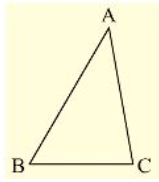We have to find longest and shortest side

We know that,

Sum of angles in a triangle 180°

• ∠ A + ∠ B + ∠ C = 180°

• 40° + 60° + ∠ C = 180°

• ∠ C = 180° – ((10)0°) = 80°

• ∠ C = 80°

Now,

=>  40° < 60° < 80° = ∠ A < ∠ B < ∠ C

=> ∠ C is greater angle and ∠ A is smaller angle.

Now, ∠ A < ∠ B < ∠ C

=> BC < AAC < AB [Side opposite to greater angle is larger and side opposite to smaller angle is smaller]

AB is longest and BC is smallest or shortest side.

Q.  (2) In a Δ ABC, if ∠ B = ∠ C = 45°, which is the longest side?

Solution: Given that in Δ ABC,

∠ B = ∠ C = 45°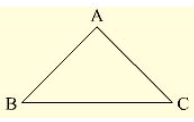We have to find longest side

We know that.

Sum of angles in a triangle =180°

• ∠ A + ∠ B + ∠ C = 180°

• ∠ A + 45° + 45° = 180°

• ∠ A = 180° – (45° + 45°) = 180° – 90° = 90°

• ∠ A = 90°

Q. (3) In Δ ABC, side AB is produced to D so that BD = BC. If ∠ B = 60° and ∠ A = 70°. prove that: (i) AD > CD (ii) AD > AC

Sol: Given that, in Δ ABC, side AB is produced to D so that BD = BC.

∠ B = 60°, and ∠ A = 70°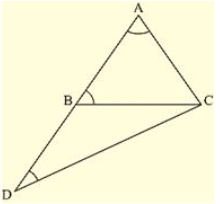To prove,

First join C and D

We know that,

Sum of angles in a triangle =180°

• ∠ A + ∠ B + ∠ C = 180°

• 70° + 60° + ∠ C = 180°

• ∠ C = 180° – (130°) = 50°

• ∠ C = 50°

• ∠ ACB = 50°……(i)

And also in Δ BDC

∠ DBC =180  – ∠ ABC [ABD is a straight angle]

• 180 – 60° = 120°

and also BD = BC[given]

• ∠ BCD = ∠ BDC [Angles opposite to equal sides are equal]

Now,

∠ DBC + ∠ BCD + ∠ BDC = 180° [Sum of angles in a triangle =180°]

=> 120° + ∠ BCD + ∠ BCD = 180°

=> 120° + 2∠ BCD = 180°

=> 2∠ BCD = 180° – 120° = 60°

=> ∠ BCD = 30°

=> ∠ BCD = ∠ BDC = 30°  ….(ii)

∠ BAC => ∠ DAC = 70° [given]

∠ BDC => ∠ ADC = 30° [From (ii)]

∠ ACD = ∠ ACB+ ∠ BCD

= 50° + 30°[From (i) and (ii)] =80°

Now, ∠ ADC < ∠ DAC < ∠ ACD

• AC < DC < AD [Side opposite to greater angle is longer and smaller angle is smaller]

Hence proved

Or,

We have,

∠ ACD > ∠ DAC and ∠ ACD > ∠ ADC

• AD > DC and AD> AC    [Side opposite to greater angle is longer and smaller angle is smaller]

Q. (4) Is it possible to draw a triangle with sides of length 2 cm, 3 cm and 7 cm?

Sol:

Given lengths of sides are 2cm, 3cm and 7cm.

To check whether it is possible to draw a triangle with the given lengths of sides

We know that,

A triangle can be drawn only when the sum of any two sides is greater than the third side.

So, let’s check the rule.

2 + 3 ≯ 7 or 2 + 3 < 7

2 + 7 > 3

and 3 + 7 > 2

Here 2 + 3 ≯ 7

So, the triangle does not exit.

Q. (5) O is any point in the interior of  Δ ABC. Prove that

(i) AB + AC > OB + OC

(ii) AB + BC + CA > OA + QB + OC

(iii) OA + OB + OC > (1/2)(AB + BC +CA)

Solution:

Given that O is any point in the interior of Δ ABC

To prove

(i) AB + AC > OB + OC

(ii) AB + BC + CA > OA + QB + OC

(iii) OA + OB + OC > (1/2)(AB + BC +CA)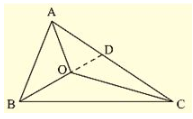We know that in a triangle the sum of any two sides is greater than the third side.

So, we have

In Δ ABC

AB + BC > AC

BC + AC > AB

AC + AB > BC

In Δ OBC

OB + OC > BC…….(i)

In Δ OAC

OA + OC > AC……(ii)

In Δ OAB

OA + OB > AB……(iii)

Now, extend (or) produce BO to meet AC in D.

Now, in Δ ABD, we have

• AB + AD > BD

• AB + AD > BO + OD …….(iv) [BD = BO + OD]

Similarly in Δ ODC, we have

OD + DC > OC……(v)

(i) Adding (iv) and (v), we get

AB + AD + OD + DC > BO + OD + OC

• AB + (AD + DC) > OB + OC

• AB + AC > OB + OC ……(vi)

Similarly, we have

BC + BA > OA + OC……(vii)

and CA+ CB > OA + OB……(viii)

(ii) Adding equation (vi), (vii) and (viii), we get

AB + AC + BC + BA + CA + CB > OB + OC + OA + OC + OA + OB => 2AB + 2BC + 2CA > 2OA + 2OB + 2OC

=> 2(AB + BC + CA) > 2(OA + OB + OC)

=> AB + BC + CA > OA + OB + OC

(iii) Adding equations (i), (ii) and (iii)

OB + OC + OA + OC + OA + OB > BC + AC + AB

• 2OA + 2OB + 2OC > AB + BC + CA

We get = 2(OA + OB + OC) > AB + BC +CA

(OA + OB + OC) > (1/2)(AB + BC +CA)

Q. (6) Prove that the perimeter of a triangle is greater than the sum of its altitudes.

Solution:

Given, Δ ABC in which AD ⊥ BC, BE ⊥ AC and CF ⊥ AB.

To prove,

AD + BE + CF < AB + BC + AC

Figure: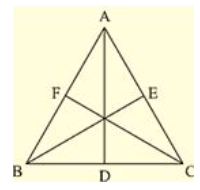Proof:

We know that of all the segments that can be drawn to a given line, from a point not lying on it, the perpendicular distance i.e, the perpendicular line segment is the shortest.

Therefore

• AB + AC > 2AD ……(i)

BE ⊥ AC

• BA > BE and BC > BE

• BA + BC > 2BE ……(ii)

CF ⊥ AB

• CA > CF and CB > CF

• CA + CB > 2CF ……(iii)

Adding (i), (ii) and (iii), we get

AB + AC + BA + BC + CA + CB > 2AD + 2BE + 2CF

• 2AB + 2BC + 2CA > 2(AD + BE + CF)

• AB + BC + CA > AD + BE + CF

• The perimeter of the triangle is greater than that the sum of its altitudes

Hence proved

Q. (7) In Fig., prove that: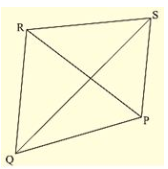(i) CD + DA + AB + BC > 2AC

(ii) CD + DA + AB > BC

Solution:

To prove

(i) CD + DA + AB + BC > 2AC

(ii) CD + DA+ AB > BC

From the given figure,

We know that, in a triangle sum of any two sides is greater than the third side

(i) So,

In Δ ABC, we have

AB + BC > AC ……(i)

CD + DA > AC……(ii)

Adding (i) and (ii), we get

AB + BC + CD + DA > AC + AC

AB + BC + CD + DA > 2AC

(ii) Now, In Δ ABC, we have,

AB +AC > BC……(iii)

And in Δ ADC, we have

CD + DA > AC

CD + DA + AB > AC + AB

From equation (iii) and (iv), we get,

CD + DA + AB > AC + AB > BC

CD + DA + AB > BC

Hence proved

Q. (8) Which of the following statements are true (T) and which are false (F)?

(i) Sum of the three sides of a triangle is less than the sum of its three altitudes.

(ii) Sum of any two sides of a triangle is greater than twice the median drown to the third side

(iii) Sum of any two sides of a triangle is greater than the third side.

(iv) Difference of any two sides of a triangle is equal to the third side.

(v) If two angles of a triangle are unequal, then the greater angle has the larger side opposite to it

(vi) Of all the line segments that can be drawn from a point to a line not containing it, the perpendicular line segment is the shortest one.

Solution:

(i) False (F)

Reason: Sum of these sides of a triangle is greater than sum of its three altitudes

(ii) True (T)

(iii) True (T)

(iv) False (F)

Reason: The difference of any two sides of a triangle is less than third side

(v) True (T)

Reason: The side opposite to greater angle is longer and smaller angle is shorter in a triangle

(vi) True (T).

Reason: The perpendicular distance is the shortest distance from a point to a line not containing it.

Q. (9) Fill in the blanks to make the following statements true.

(i) In a right triangle the hypotenuse is the ___ side.

(ii) The sum of three altitudes of a triangle is ___ than its perimeter.

(iii) The sum of any two sides of a triangle is ___ than the third side.

(iv) If two angles of a triangle are unequal, then the smaller angle has the ___ side opposite to it.

(v) Difference of any two sides of a triangle is ___ than the third side.

(vi) If two sides of a triangle are unequal, then the larger side has ___ angle opposite to it.

Solution:

(i) In a right triangle the hypotenuse is the largest side

Reason: Since a triangle can have only one right angle, other two angles must be less than 90

• The right angle is the largest angle.

• Hypotenuse is the largest side.

(ii) The sum of three altitudes of a triangle is less than its perimeter.

(iii) The sum of any two sides of a triangle is greater than the third side. (iv) If two angles of a triangle are unequal, then the smaller angle has the smaller side opposite to it.

(v) Difference of any two sides of a triangle is less than the third side.

(vi) If two sides of a triangle are unequal, then the larger side has greater angle opposite to it.

Offer running on EduRev: Apply code STAYHOME200 to get INR 200 off on our premium plan EduRev Infinity!

91 docs

,

,

,

,

,

,

,

,

,

,

,

,

,

,

,

,

,

,

,

,

,

,

,

,

,

,

,

,

,

,

;